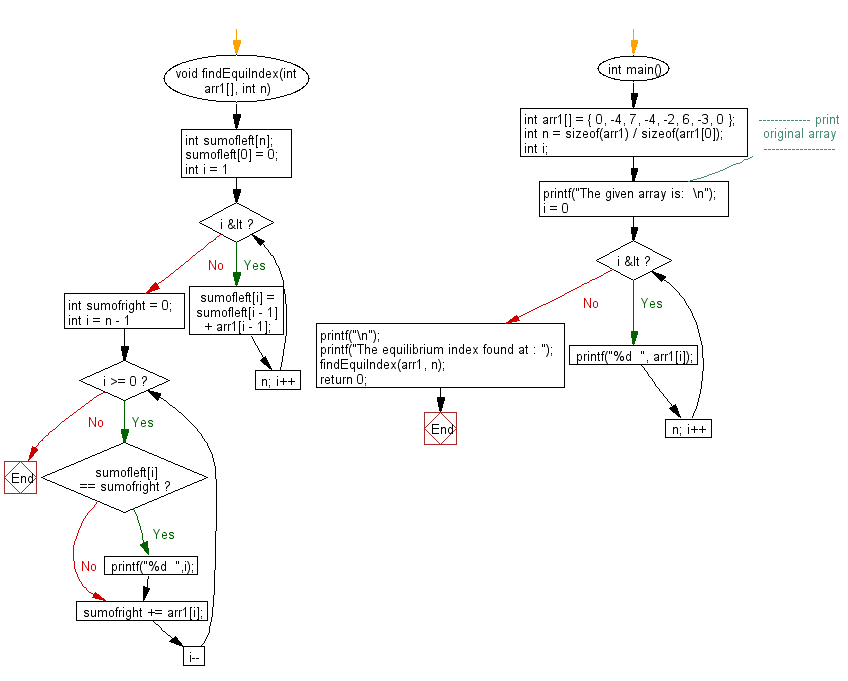﻿ C exercises: Find the equilibrium index of an array - w3resource# C Exercises: Find the equilibrium index of an array

## C Array: Exercise-86 with Solution

Write a program in C to find the equilibrium index of an array.

Sample Solution:

C Code:

``````#include <stdio.h>

void findEquiIndex(int arr1[], int n)
{
int sumofleft[n];
sumofleft = 0;
for (int i = 1; i < n; i++)
{
sumofleft[i] = sumofleft[i - 1] + arr1[i - 1];
}
int sumofright = 0;
for (int i = n - 1; i >= 0; i--)
{
if (sumofleft[i] == sumofright)
{
printf("%d  ",i);
}
sumofright += arr1[i];
}
}

int main()
{
int arr1[] = { 0, -4, 7, -4, -2, 6, -3, 0 };
int n = sizeof(arr1) / sizeof(arr1);
int i;
//------------- print original array ------------------
printf("The given array is:  \n");
for(i = 0; i < n; i++)
{
printf("%d  ", arr1[i]);
}
printf("\n");
//-----------------------------------------------------------
printf("The equilibrium index found at : ");
findEquiIndex(arr1, n);
return 0;
}
```
```

Sample Output:

```The given array is:
0  -4  7  -4  -2  6  -3  0
The equilibrium index found at : 7  5  0
```

Pictorial Presentation:Flowchart:C Programming Code Editor:

Improve this sample solution and post your code through Disqus.

﻿